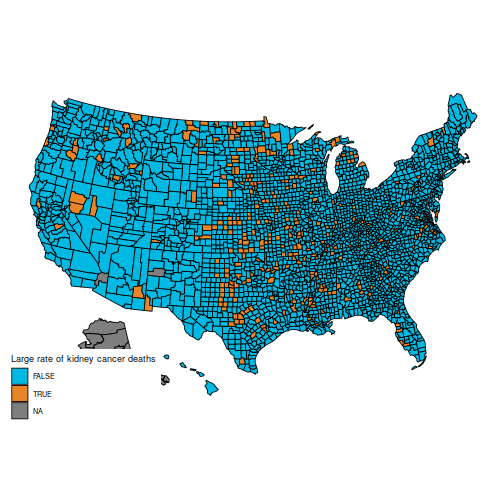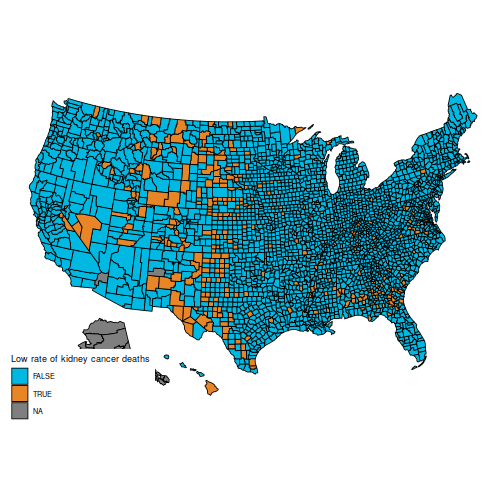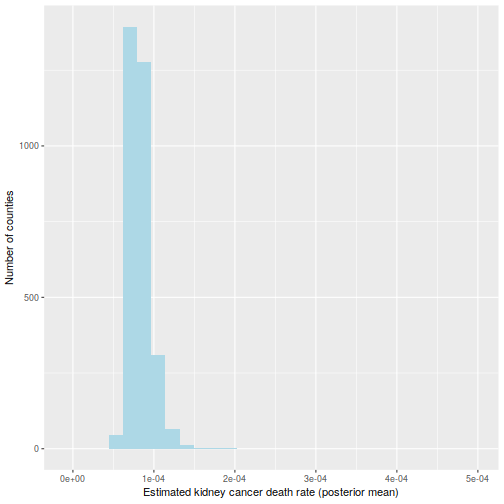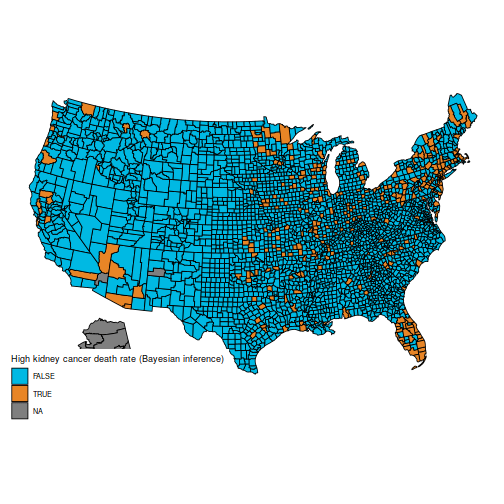Want to share your content on R-bloggers? click here if you have a blog, or here if you don't.

This is an attempt at reproducing the analysis of Section 2.7 of Bayesian Data Analysis, 3rd edition (Gelman et al.), on kidney cancer rates in the USA in the 1980s. I have done my best to clean the data from the original. Andrew wrote a blog post to “disillusion [us] about the reproducibility of textbook analysis”, in which he refers to this example. This might then be an attempt at reillusionment…

The cleaner data are on GitHub, as is the RMarkDown of this analysis.

library(usmap)
library(ggplot2)



In the data, the columns dc and dc.2 correspond (I think) to the death counts due to kidney cancer in each county of the USA, respectively in 1908-84 and 1985-89. The columns pop and pop.2 are some measure of the population in the counties. It is not clear to me what the other columns represent.

## Simple model

Let$n_j$ be the population on county$j$, and$K_j$ the number of kidney cancer deaths in that county between 1980 and 1989. A simple model is$K_j\sim Poisson(\theta_j n_j)$ where$\theta_j$ is the unknown parameter of interest, representing the incidence of kidney cancer in that county. The maximum likelihood estimator is$\hat\theta_j=\frac{K_j}{n_j}$.

d$dct = d$dc + d$dc.2 d$popm = (d$pop + d$pop.2) / 2
d$thetahat = d$dct / d$popm  In particular, the original question is to understand these two maps, which show the counties in the first and last decile for kidney cancer deaths. q = quantile(d$thetahat, c(.1, .9))
d$cancerlow = d$thetahat <= q d$cancerhigh = d$thetahat >= q
plot_usmap("counties", data=d, values="cancerhigh") +
scale_fill_discrete(h.start = 200,
name = "Large rate of kidney cancer deaths")plot_usmap("counties", data=d, values="cancerlow") +
scale_fill_discrete(h.start = 200,
name = "Low rate of kidney cancer deaths")These maps are suprising, because the counties with the highest kidney cancer death rate, and those with the lowest, are somewhat similar: mostly counties in the middle of the map.

(Also, note that the data for Alaska are missing. You can hide Alaska on the maps by adding the parameter include = statepop$full[-2] to calls to plot_usmap.) The reason for this pattern (as explained in BDA3) is that these are counties with a low population. Indeed, a typical value for$\hat\theta_j$ is around$0.0001$. Take a county with a population of 1000. It is likely to have no kidney cancer deaths, giving$\hat\theta_j=0$ and putting it in the first decile. But if it happens to have a single death, the estimated rate jumps to$\hat\theta_j=0.001$ (10 times the average rate), putting it in the last decile. This is hinted at in this histogram of the$(\theta_j)$: ggplot(data=d, aes(d$thetahat)) +
geom_histogram(bins=30, fill="lightblue") +
labs(x="Estimated kidney cancer death rate (maximum likelihood)",
y="Number of counties") +
xlim(c(-1e-5, 5e-4))## Bayesian approach

If you have ever followed a Bayesian modelling course, you are probably screaming that this calls for a hierarchical model. I agree (and I’m pretty sure the authors of BDA do as well), but here is a more basic Bayesian approach. Take a common$\Gamma(\alpha, \beta)$ distribution for all the$(\theta_j)$; I’ll go for$\alpha=15$ and$\beta = 200\ 000$, which is slightly vaguer than the prior used in BDA. Obviously, you should try various values of the prior parameters to check their influence.

The prior is conjugate, so the posterior is$\theta_j|K_j \sim \Gamma(\alpha + K_j, \beta + n_j)$. For small counties, the posterior will be extremely close to the prior; for larger counties, the likelihood will take over.

It is usually a shame to use only point estimates, but here it will be sufficient: let us compute the posterior mean of$\theta_j$. Because the prior has a strong impact on counties with low population, the histogram looks very different:

alpha = 15
beta = 2e5
d$thetabayes = (alpha + d$dct) / (beta + d$pop) ggplot(data=d, aes(d$thetabayes)) +
geom_histogram(bins=30, fill="lightblue") +
labs(x="Estimated kidney cancer death rate (posterior mean)",
y="Number of counties") +
xlim(c(-1e-5, 5e-4))And the maps of counties in the first and last decile are now much easier to distinguish; for instance, Florida and New England are heavily represented in the last decile. The counties represented here are mostly populated counties: these are counties for which we have reason to believe that they are on the lower or higher end for kidney cancer death rates.

qb = quantile(d$thetabayes, c(.1, .9)) d$bayeslow = d$thetabayes <= qb d$bayeshigh = d\$thetabayes >= qb
plot_usmap("counties", data=d, values="bayeslow") +
scale_fill_discrete(
h.start = 200,
name = "Low kidney cancer death rate (Bayesian inference)")plot_usmap("counties", data=d, values="bayeshigh") +
scale_fill_discrete(
h.start = 200,
name = "High kidney cancer death rate (Bayesian inference)")An important caveat: I am not an expert on cancer rates (and I expect some of the vocabulary I used is ill-chosen), nor do I claim that the data here are correct (from what I understand, many adjustments need to be made, but they are not detailed in BDA, which explains why the maps are slightly different). I am merely posting this as a reproducible example where the naïve frequentist and Bayesian estimators differ appreciably, because they handle sample size in different ways. I have found this example to be useful in introductory Bayesian courses, as the difference is easy to grasp for students who are new to Bayesian inference.